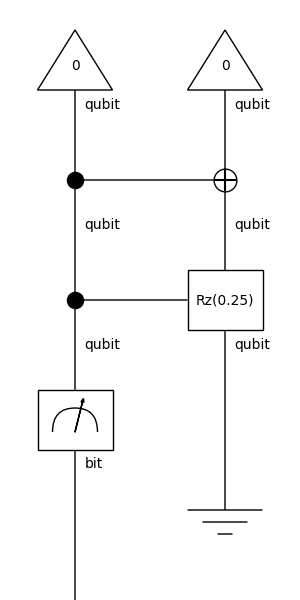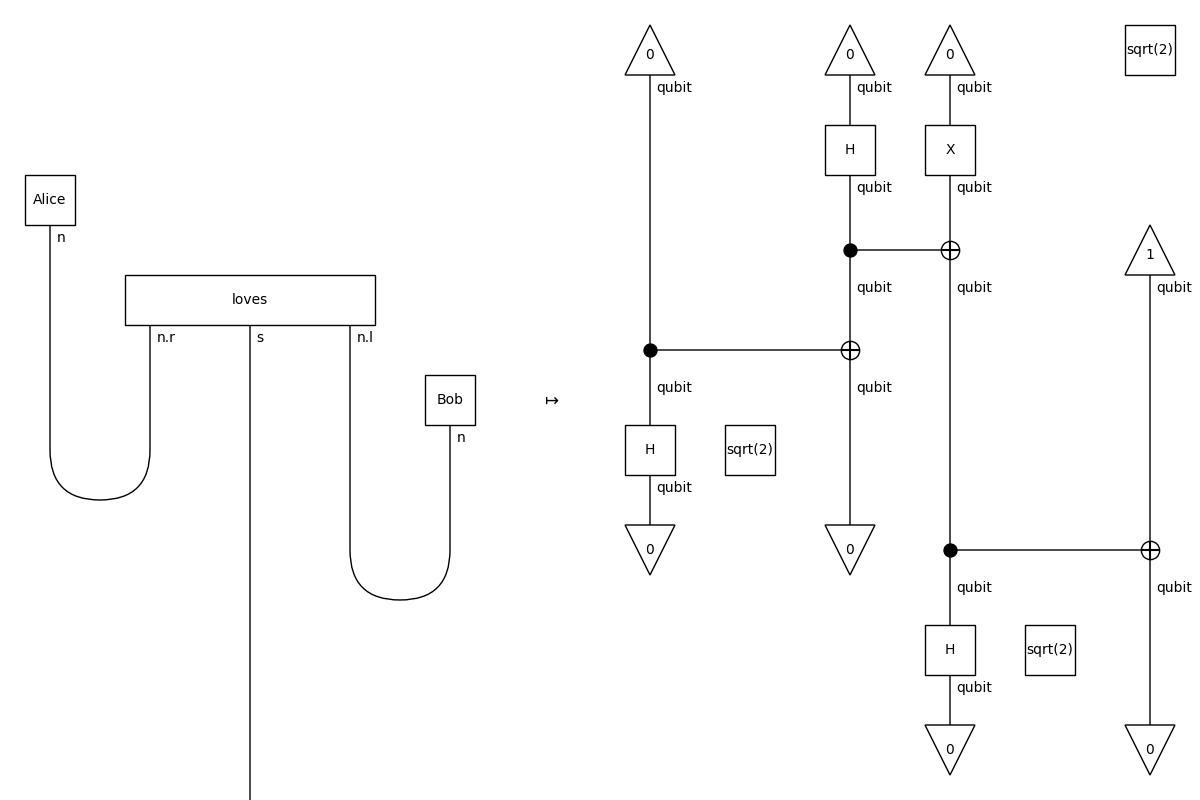# circuit#

The category of classical-quantum circuits with digits and qudits as objects.

## Summary#

 `Ob` A circuit object is an information unit with some dimension `dim > 1`. `Digit` A digit is a classical unit of information. `Qudit` A qudit is a quantum unit of information, i.e. a quantum digit. `Ty` A circuit type is a frobenius type with `Digit` and `Qudit` objects inside. `Circuit` A circuit is a tensor diagram with bits and qubits as `dom` and `cod`. `Box` A circuit box is a tensor box in a circuit diagram. `Sum` Sums of circuits. `Swap` Implements swaps of circuit wires. `Functor` `Circuit`-valued functor.

Functions

 `index2bitstring` Turns an index into a bitstring of a given length. `bitstring2index` Turns a bitstring into an index.

Examples

```>>> from discopy.quantum.gates import (
...     Ket, CX, H, X, Rz, sqrt, Controlled, Measure, Discard)
>>> circuit = Ket(0, 0) >> CX >> Controlled(Rz(0.25)) >> Measure() @ Discard()
>>> circuit.draw(
...     figsize=(3, 6),
...     path='docs/_static/quantum/circuit-example.png')
``````>>> from discopy.grammar import pregroup
>>> s, n = pregroup.Ty('s'), pregroup.Ty('n')
>>> Alice = pregroup.Word('Alice', n)
>>> loves = pregroup.Word('loves', n.r @ s @ n.l)
>>> Bob = pregroup.Word('Bob', n)
>>> grammar = pregroup.Cup(n, n.r) @ s @ pregroup.Cup(n.l, n)
>>> sentence = grammar << Alice @ loves @ Bob
>>> ob = {s: Ty(), n: qubit}
>>> ar = {Alice: Ket(0),
...       loves: CX << sqrt(2) @ H @ X << Ket(0, 0),
...       Bob: Ket(1)}
>>> F = pregroup.Functor(ob, ar, cod=Category(Ty, Circuit))
>>> assert abs(F(sentence).eval().array) ** 2
```
```>>> from discopy.drawing import Equation
>>> Equation(
...     sentence.to_drawing(), F(sentence), symbol='\$\\mapsto\$').draw(
...         figsize=(6, 3), nodesize=.5,
...         path='docs/_static/quantum/functor-example.png')
```